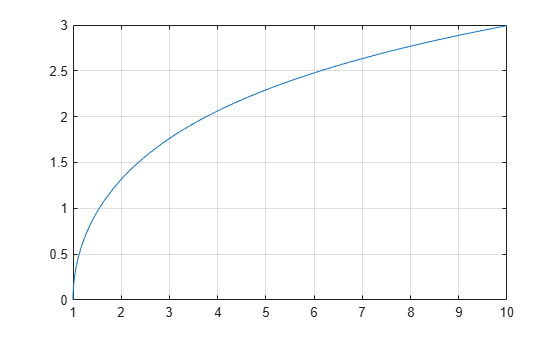# acosh

Symbolic inverse hyperbolic cosine function

## Syntax

``acosh(X)``

## Description

example

````acosh(X)` returns the inverse hyperbolic cosine function of `X`.```

## Examples

### Inverse Hyperbolic Cosine Function for Numeric and Symbolic Arguments

Depending on its arguments, `acosh` returns floating-point or exact symbolic results.

Compute the inverse hyperbolic cosine function for these numbers. Because these numbers are not symbolic objects, `acosh` returns floating-point results.

`A = acosh([-1, 0, 1/6, 1/2, 1, 2])`
```A = 0.0000 + 3.1416i 0.0000 + 1.5708i 0.0000 + 1.4033i... 0.0000 + 1.0472i 0.0000 + 0.0000i 1.3170 + 0.0000i```

Compute the inverse hyperbolic cosine function for the numbers converted to symbolic objects. For many symbolic (exact) numbers, `acosh` returns unresolved symbolic calls.

`symA = acosh(sym([-1, 0, 1/6, 1/2, 1, 2]))`
```symA = [ pi*1i, (pi*1i)/2, acosh(1/6), (pi*1i)/3, 0, acosh(2)]```

Use `vpa` to approximate symbolic results with floating-point numbers:

`vpa(symA)`
```ans = [ 3.1415926535897932384626433832795i,... 1.5707963267948966192313216916398i,... 1.4033482475752072886780470855961i,... 1.0471975511965977461542144610932i,... 0,... 1.316957896924816708625046347308]```

### Plot Inverse Hyperbolic Cosine Function

Plot the inverse hyperbolic cosine function on the interval from 1 to 10.

```syms x fplot(acosh(x),[1 10]) grid on```### Handle Expressions Containing Inverse Hyperbolic Cosine Function

Many functions, such as `diff`, `int`, `taylor`, and `rewrite`, can handle expressions containing `acosh`.

Find the first and second derivatives of the inverse hyperbolic cosine function. Simplify the second derivative by using `simplify`.

```syms x diff(acosh(x), x) simplify(diff(acosh(x), x, x))```
```ans = 1/((x - 1)^(1/2)*(x + 1)^(1/2)) ans = -x/((x - 1)^(3/2)*(x + 1)^(3/2))```

Find the indefinite integral of the inverse hyperbolic cosine function. Simplify the result by using `simplify`.

`int(acosh(x), x)`
```ans = x*acosh(x) - (x - 1)^(1/2)*(x + 1)^(1/2)```

Find the Taylor series expansion of `acosh(x)` for ```x > 1```:

```assume(x > 1) taylor(acosh(x), x)```
```ans = (x^5*3i)/40 + (x^3*1i)/6 + x*1i - (pi*1i)/2```

For further computations, clear the assumption on `x` by recreating it using `syms`:

`syms x`

Rewrite the inverse hyperbolic cosine function in terms of the natural logarithm:

`rewrite(acosh(x), 'log')`
```ans = log(x + (x - 1)^(1/2)*(x + 1)^(1/2))```

## Input Arguments

collapse all

Input, specified as a symbolic number, variable, expression, or function, or as a vector or matrix of symbolic numbers, variables, expressions, or functions.